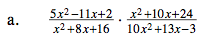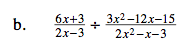### Home > CAAC > Chapter 10 > Lesson 10.1.4 > Problem10-39

10-39.

For more help with multiplying and dividing fractional expressions, see problem 10-30.Factor the expressions and multiply the two fractions.

Simplify by looking for factors from the numerator and the denominator that make 'one'.

$\frac{(x-2)(5x-1)(x+6)(x+4)}{(x+4)(x+4)(2x+3)(5x-1)}$$\frac{2x+1}{x-5}$